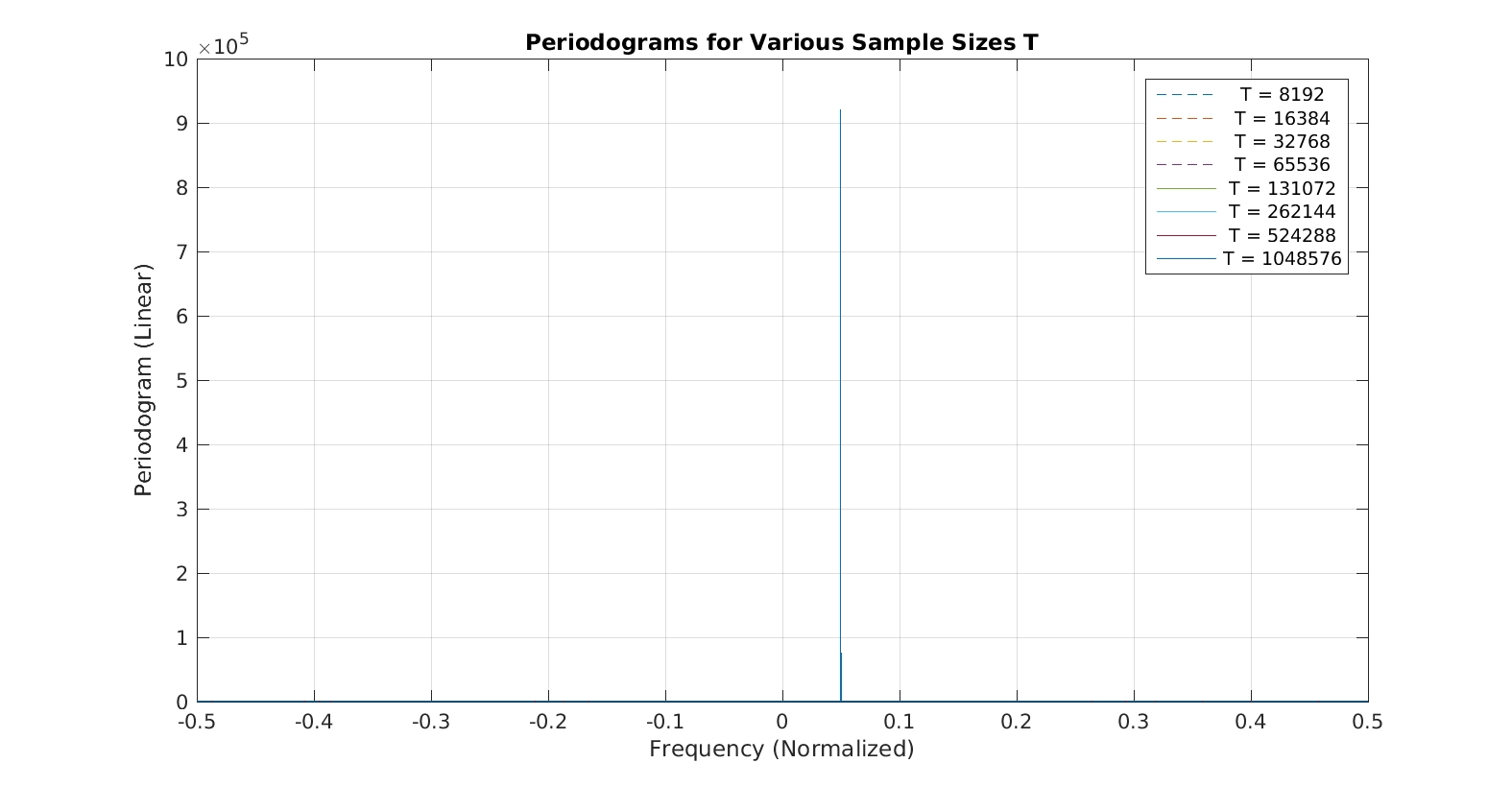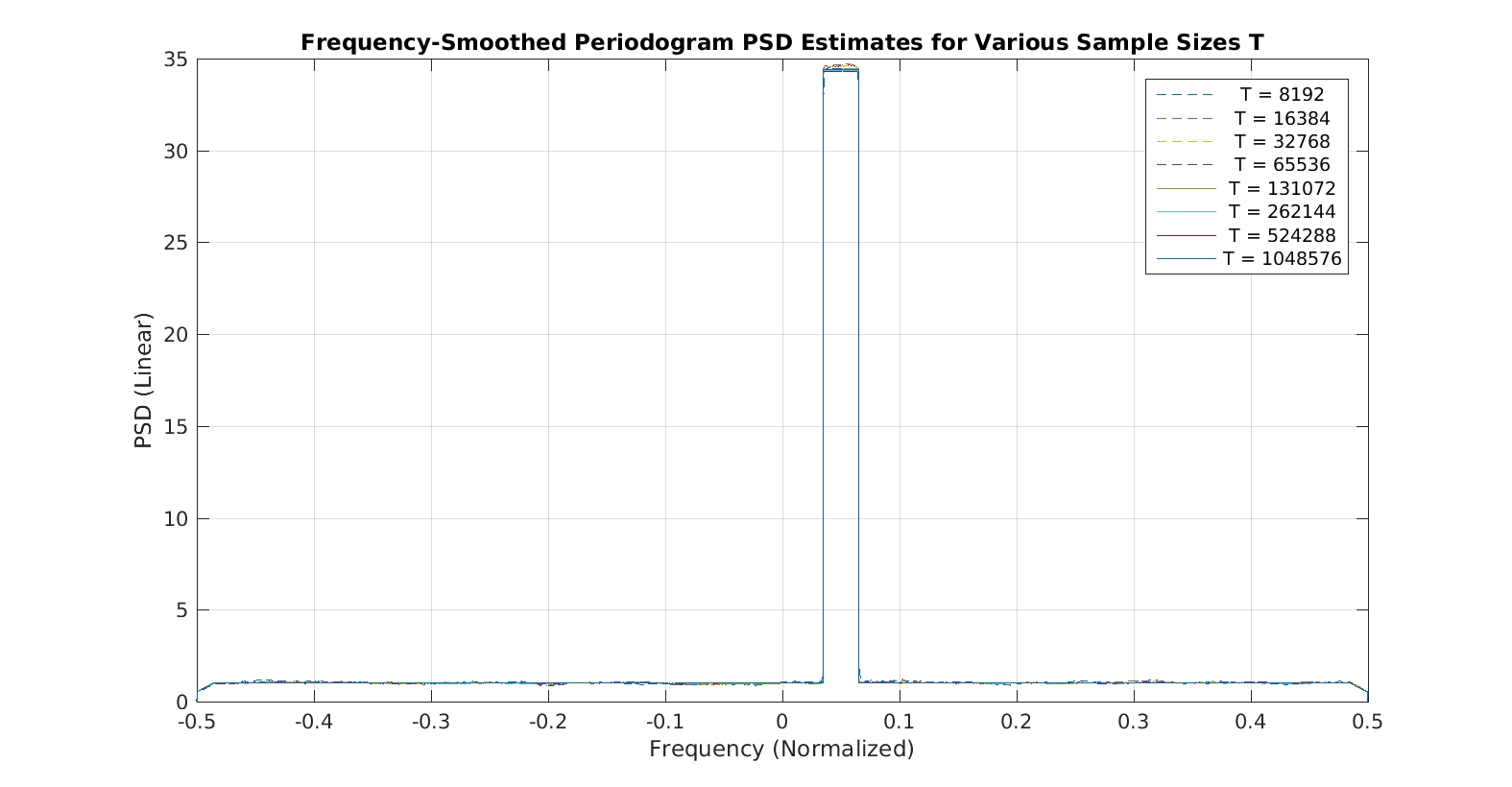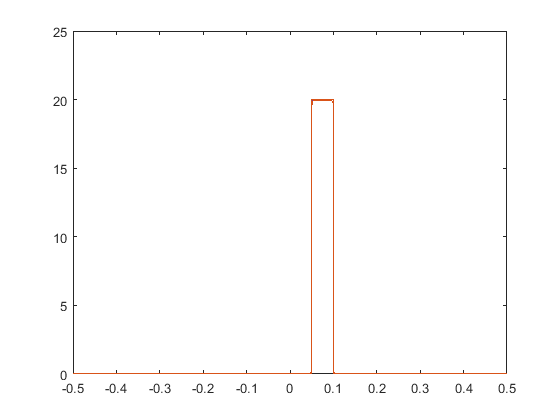# The Periodogram

The periodogram is the scaled magnitude-squared finite-time Fourier transform of something. It is as random as its input–it never converges to the power spectrum.

I’ve been reviewing a lot of technical papers lately and I’m noticing that it is becoming common to assert that the limiting form of the periodogram is the power spectral density or that the limiting form of the cyclic periodogram is the spectral correlation function. This isn’t true. These functions do not become, in general, less random (erratic) as the amount of data that is processed increases without limit. On the contrary, they always have large variance. Some form of averaging (temporal or spectral) is needed to permit the periodogram to converge to the power spectrum or the cyclic periodogram to converge to the spectral correlation function (SCF).

In particular, I’ve been seeing things like this:$\displaystyle S_x^\alpha(f) = \lim_{T\rightarrow\infty} \frac{1}{T} X_T(f+\alpha/2) X_T^*(f-\alpha/2), \hfill (1)$

where$X_T(f+\alpha/2)$ is the Fourier transform of$x(t)$ on$t \in [-T/2, T/2]$. In other words, the usual cyclic periodogram we talk about here on the CSP blog. See, for example, The Literature [R71], Equation (3).

Similarly, I see asserted:$\displaystyle S_x^0(f) = S_x(f) = \lim_{T\rightarrow\infty} \frac{1}{T} \left| X_T(f) \right|^2. \hfill (2)$

In (1), the quantity$\displaystyle \frac{1}{T} X_T(f+\alpha/2) X_T^*(f-\alpha/2) \hfill (3)$

is the non-conjugate cyclic periodogram, and in (2), the quantity$\displaystyle \frac{1}{T} \left| X_T(f) \right|^2 \hfill (4)$

is the conventional periodogram. The conjugate cyclic periodogram is given by$\displaystyle \frac{1}{T} X_T(f+\alpha/2) X_T(\alpha/2 - f). \hfill (5)$

But (1) and (2) are not true. Standard textbooks on spectral analysis typically establish the fact that the asymptotic ($T$ is large) variance of the periodogram for random inputs is equal to the square of the true power spectral density (PSD) value. See The Literature [R70] for example.

If the periodogram is smoothed over frequency, say by convolving it with a pulse-like function$g_{\Delta}(f)$ having unit area, then the theoretical spectral correlation function can be obtained by first letting the Fourier transform length (the data-block length)$T$ approach$\infty$, and then letting the width of the smoothing function$\Delta$ approach zero, in that order. We discussed this in previous posts, see also The Literature [R1].

What follows is some numerical evidence for my assertions above. First, let’s just look at the periodogram ($\alpha = 0$, non-conjugate SCF) and the frequency-smoothed periodogram estimate of the power spectrum. The data is a simulated textbook BPSK signal in additive white Gaussian noise. The BPSK signal has a bit rate of$1/5 = 0.2$ (I’m using normalized frequencies here, as usual on the CSP Blog), and a carrier offset frequency of$0.05$. The pulse function is rectangular (similar to, but not identical to, our old friend), the signal power is unity and the noise spectral density is also unity. I show below a sequence of graphs that correspond to successively longer processed data blocks, with length$T$ samples, drawn from a single very long simulated data file. Each data block is taken from the data file starting with the first sample, so that, for example, all the data points for$T=8192$ are also included in the data block for$T=16384$. So this set-up is consistent with the right sides of (1) and (2) above.

### Periodogram and Frequency-Smoothed Periodogram PSD Estimate (FSM)

First the periodogram:Figure 1. Periodograms for various data-block lengths T for a rectangular-pulse BPSK signal with rate 0.2 and carrier 0.05.

which looks quite erratic, and which has a higher variance where the PSD is larger (near$0.05$), as expected. If we zoom in near zero frequency, we obtain the following plot:Figure 2. Periodograms for various data-block lengths T for a rectangular-pulse BPSK signal with rate 0.2 and carrier 0.05. (Zoomed version of Figure 1.)

which clearly shows that the sequence of periodograms is not converging as$T$ increases. On the other hand, the frequency-smoothed periodograms result in power-spectrum estimates that do converge as$T$ grows:Figure 3. Power spectrum estimates for various data-block lengths T for a rectangular-pulse BPSK signal with rate 0.2 and carrier 0.05. The PSDs are obtained using frequency-smoothed periodograms.

Not only do they converge, but the erratic nature of the estimate decreases with increasing$T$.

### Non-Conjugate Cyclic Periodogram and Frequency-Smoothed Cyclic Periodogram (SCF)

Now here are the non-conjugate cyclic periodogram magnitudes for a non-conjugate cycle frequency equal to the BPSK bit rate of$0.2$:Figure 4. Non-conjugate cyclic periodograms for various data-block lengths T for a rectangular-pulse BPSK signal with rate 0.2 and carrier 0.05. The cycle frequency is the BPSK bit rate.

and its zoomed version:Figure 5. Non-conjugate cyclic periodograms for various data-block lengths T for a rectangular-pulse BPSK signal with rate 0.2 and carrier 0.05. The cycle frequency is the BPSK bit rate. (Zoomed version of Figure 4.)

Again, highly erratic and no convergence. Here is the frequency-smoothed non-conjugate cyclic periodogram for the bit-rate cycle frequency:Figure 6. Non-conjugate spectral correlation function estimates for various data-block lengths T for a rectangular-pulse BPSK signal with rate 0.2 and carrier 0.05. The cycle frequency is the BPSK bit rate.

### Conjugate Cyclic Periodogram and Frequency-Smoothed Cyclic Periodogram (SCF)

Finally, for completeness, let’s do the same thing for the conjugate cyclic periodogram and conjugate spectral correlation function, using the BPSK doubled-carrier cycle frequency equal to$0.1$:Figure 7. Conjugate cyclic periodograms for various data-block lengths T for a rectangular-pulse BPSK signal with rate 0.2 and carrier 0.05. The cycle frequency is the BPSK doubled carrier frequency.Figure 8. Conjugate cyclic periodograms for various data-block lengths T for a rectangular-pulse BPSK signal with rate 0.2 and carrier 0.05. The cycle frequency is the BPSK doubled carrier frequency. (Zoomed version of Figure 7.)Figure 9. Conjugate spectral correlation function estimates for various data-block lengths T for a rectangular-pulse BPSK signal with rate 0.2 and carrier 0.05. The cycle frequency is the BPSK doubled carrier frequency.

### What is the Periodogram Good For?

It is a good detector for periodic signals such as sine waves. The Fourier transform is representing its input in terms of the complex-valued coefficients of basis functions that are complex sine-waves, so when the input data contains a sine-wave component, a single Fourier coefficient (we’re neglecting spectral leakage here) becomes very large and the rest are small. So even when the periodic function is embedded in random noise, a simple peak detector applied to the periodogram can easily find the signal, leading to good amplitude, phase, and frequency estimation. For example, if we repeat the experiment above by replacing the BPSK signal with a tone, we obtain the following periodograms and power spectra:Figure 10. Periodograms for a noisy sine-wave input for various data-block sizes T. The frequency of the sine wave is 0.05.Figure 11. Power spectrum estimates for a noisy sine-wave input for various data-block sizes T. The frequency of the sine wave is 0.05. The frequency-smoothed periodogram method is used to estimate the PSDs.

The rectangular appearance of the impulse arising from the tone is due to the shape of the frequency-smoothing window$g_{\Delta}(f)$ I used, which is a rectangle.

So that’s it. I hope it is clear that the periodogram and the cyclic periodogram do not converge to the power spectrum and spectral correlation functions, respectively, no matter how much data is processed when we are dealing with random signals and noise such as those encountered in communication systems.

As always, let me know if you see any errors in the post, disagree, or have links to relevant websites.I'm a signal processing researcher specializing in cyclostationary signal processing (CSP) for communication signals. I hope to use this blog to help others with their cyclo-projects and to learn more about how CSP is being used and extended worldwide.

Posted on

## 7 thoughts on “The Periodogram”

1.Mimi says:

I was trying to produce the Periodogram plot for rectangular BPSK signal (I used your code make_rect_bpsk to generate rectangular BPSK signal with white Gaussian noise). I created a long signal of x=1048576*5 samples.

Then I took different number of samples (T=8192, 16384, … 1048576) from this signal as x1=x(1:T). Then I applied FFT on x1.

x1_FT=abs(fft(x1))/T;
Then I plotted (fftshift(x1_FT)) against normalized frequency.

freq=(-length(x_FT)/2+1:length(x_FT)/2)/(length(x_FT));
plot(freq,10*log10(fftshift(x_FT)));hold on;

But my plot is not same as yours.

1.Chad Spooner says:

Thanks for checking out the CSP Blog, Mimi, and for the comment! I really appreciate it.

(I used your code make_rect_bpsk to generate rectangular BPSK signal with white Gaussian noise).

The signal in the Periodogram post is similar to the one from many of my other posts, which are generated by the code I posted, but the bit rate is 0.2 in the Periodogram post, not 0.1. Did you adjust the downloaded code?

x1_FT=abs(fft(x1))/T;
Then I plotted (fftshift(x1_FT)) against normalized frequency.

x1_FT is not the periodogram. Equation (4) provides the periodogram definition–you must square the magnitude of the Fourier transform and scale it by the data-block length.

I’ve modified the post to include figure numbers and figure captions. If you still have trouble, let me know which figure(s) you are trying to replicate and we’ll take it from there.

1.Mimi says:

Thanks for all the help. It is great that you are so prompt to reply to help others.

I was trying to replicate Figure 1 and 3 of this post to see the difference the Frequency Smoothing does on Periodogram. I used your make_rect_bpsk.m code to generate y_t (exact code, just changed the T_bit to 5 (to make bit rate 0.2). Then I did the following:

% x=y_t;
x=exp(sqrt(-1)*2*pi*0.05*t); %single tone of frequency 0.05
G_f=ones(1,1*Fs/1000); % Frequency domain G(f) for frequency smoothing

for pow=13:20
x1=x(1:2^pow); % For T=2^13 to T^20
x_FT=fft(x1);
x_FT = x_FT .* conj(x_FT);
x_FT = x_FT /(length(x_FT));
G_f=ones(1,round(length(x_FT)/20));
G_f=G_f/length(G_f);
x_FT_smoothed=filter(G_f,,x_FT);
x_FT=fftshift(x_FT);
axis_freq=(-length(x_FT)/2+1:length(x_FT)/2)/(length(x_FT));
figure(1);plot(axis_freq,(x_FT));hold on;
x_FT_smoothed=fftshift(x_FT_smoothed);
figure(2);plot(axis_freq,(x_FT_smoothed));hold on;
pause
end

For the single tone, it produces the same figure as in Figure 10, But for Figure 11, you got the 0.5 point in the middle of the rect, for me 0.05 is at the leading edge of the rect. I used filter function and thought the shifting is done by itself.

For x=y_t, I get the figures not similar as Figure 1 and 3. For Figure 3, it has a dent at freq=0, there may be a division by zero, do I need to fix it manually?1.Chad Spooner says:

You are almost there.

1. If you use MATLAB’s filter.m to do the frequency smoothing, you must compensate for the filter delay. That is why your rectangle is shifted with respect to mine.

2. You should apply fftshift.m before you do the smoothing, not after. In fact, apply it just after computing the FFT. That will remove your null at f=0.

1.Mimi says:

That helps. Now I understand what you mentioned about the shifting of X(f) and then multiplying.

2.Mimi says:

I got the two different implementation for the SCF and the output.
—————————————————————
The first implementation is FT[x(t)*exp(-j*2*pi*alpha/2*t)] * conj{FT[x(t)*exp(j*2*pi*alpha/2*t)]}. I am not sure why the non conjugate SCF is upside down and the conjugate SCF is not working at all.

x=y_t;
% x=exp(sqrt(-1)*2*pi*0.05*t); %single tone of frequency 0.05

alpha=0.2;

for pow=13:20
x1=x(1:2^pow).*exp(-sqrt(-1)*2*pi*alpha/2.*[1:2^pow]); % For T=2^13 to T^20
x2=x(1:2^pow).*exp(sqrt(-1)*2*pi*alpha/2.*[1:2^pow]);
x1_FT=fft(x1);
x1_FT=fftshift(x1_FT);
x2_FT=fft(x2);
x2_FT=fftshift(x2_FT);
cyclic_PSD = -x1_FT .* conj(x2_FT);
cyclic_PSD = cyclic_PSD /(length(cyclic_PSD));
G_f=ones(1,round(length(cyclic_PSD)/50));
G_f=G_f/length(G_f);
cyclic_PSD_smoothed=conv(cyclic_PSD,G_f,’same’);
axis_freq=(-length(cyclic_PSD)/2+1:length(cyclic_PSD)/2)/(length(cyclic_PSD));
figure(1);plot(axis_freq,(cyclic_PSD));hold on;
figure(2);plot(axis_freq,(cyclic_PSD_smoothed));hold on;
%——————————————————
conj_cyclic_PSD = x1_FT .* (x2_FT);
conj_cyclic_PSD = conj_cyclic_PSD /(length(conj_cyclic_PSD));
conj_cyclic_PSD_smoothed=conv(conj_cyclic_PSD,G_f,’same’);
figure(3);plot(axis_freq,(conj_cyclic_PSD));hold on;
figure(4);plot(axis_freq,(conj_cyclic_PSD_smoothed));hold on;
pause
end
—————————————————————————-
The second implementation is FT(x(t))=X(f). Then X(f+alfa/2).*conj(X(f-alfa/2)). This is not working at all.

x=y_t;
% x=exp(sqrt(-1)*2*pi*0.05*t); %single tone of frequency 0.05

Fs=1000000; % Sampling Frequency is 1 MHz
alpha=0.2*Fs;

for pow=13:20
x1=x(1:2^pow+alpha); % For T=2^13 to T^20
x_FT=fft(x1);
x_FT=fftshift(x_FT);

X_down_alfa_2=x_FT(1:2^pow);
X_up_alfa_2=x_FT(alpha+1:2^pow+alpha);
x_cyclic_FT = X_down_alfa_2 .* (X_up_alfa_2);
x_cyclic_FT = x_cyclic_FT /(length(x_cyclic_FT));
G_f=ones(1,round(length(x_cyclic_FT)/20));
G_f=G_f/length(G_f);
x_cyclic_FT_smoothed=conv(x_cyclic_FT,G_f,’same’);
axis_freq=(-length(x_cyclic_FT)/2+1:length(x_cyclic_FT)/2)/(length(x_cyclic_FT));
figure(1);plot(axis_freq,(x_cyclic_FT));hold on;
figure(2);plot(axis_freq,(x_cyclic_FT_smoothed));hold on;
pause
end

3.Chad Spooner says:

Your first method appears to work fine–you just need to plot the magnitude of the SCF estimates, so include ‘abs()’.

The second method has some problems. I don’t think you should add alpha to x in

x1=x(1:2^pow+alpha); % For T=2^13 to T^20

When you are attempting to shift the FFT output,

X_up_alfa_2=x_FT(alpha+1:2^pow+alpha);

you are shifting it with ‘alpha’, which you define in Hz as 0.2*Fs. You want to convert the shift into an equivalent number of FFT bins.